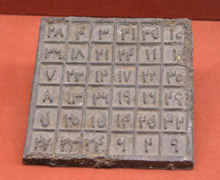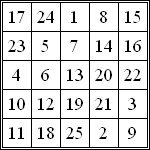A# magic square

A magic square is an n × n square of the distinct whole numbers 1, 2, ..., n 2, such that the sum of the numbers along any row, column, or main diagonal is the same. This sum is known as the magic constant and is equal to ½n(n 2 + 1). There is only one 3 × 3 magic square (not counting reflections and rotations), which was known to the Chinese as long ago as 650 BC as lo-shu and is bound up with a variety of myths. Associations between magic squares and the supernatural are also evident in early Indian and Arabian mathematics. The 3 × 3 square can be written as:

 8 1 6 3 5 7 4 9 2

Each row, column, and main diagonal sums to 15. If the rows are read as three-digit numbers, forwards and backwards, and then squared, we find the interesting relation

8162 + 3572 + 4922 = 6182 + 7532 + 2942.

The reader may wish to see if the same rule holds for the columns and main diagonals.An old Chinese magic square

In the early 16th century Cornelius Agrippa constructed squares for n = 3, 4, 5, 6, 7, 8, and 9, which he associated with the seven "planets" then known (including the Sun and the Moon). Albrecht Dürer's famous engraving of Melancholia (1514) includes a picture of an order-4 magic square. There are 880 distinct squares of order-4 and 275,305,224 squares of order-5, but the number of larger squares is unknown. A square that fails to be magic only because one or both of the main diagonal sums don't equal the magic constant is called a semi-magic square. If all diagonals (including those obtained by wrapping around) of a magic square sum to the magic constant, the square is said to be a pandiagonal square (also known as a panmagic or diabolical square). Pandiagonal squares exist for all orders except 6, 10, 14, ..., 2(2i + 1). There are 48 pandiagonal 4 × 4 squares. If replacing each number ni by its square ni2 produces another magic square, the square is said to be a bimagic or doubly magic square. If a square is magic for ni, ni2, and ni3, it is known as a trebly magic square.A magic square of order 5

A little trial and improvement is all it takes to construct the 3 × 3 magic square, but for building 4 × 4 squares and larger, a systematic method, or algorithm, is important. Interestingly, different algorithms are needed depending in whether the square is of an even order or an odd order. Odd order squares are the easier variety to make and there are several standard techniques, including the Siamese (sometimes called de la Loubere's or the Staircase), the Lozenge, and de Meziriac's methods. Here is yet another approach, known as the Pyramid or extended diagonals method: (1) Draw a pyramid of same size squares as the magic square's squares, on each side of the magic square; the pyramid should be two less, in number of squares on its base, than the number of squares on the side of the magic square. (2) Sequentially place the numbers 1 to n 2 of the n × n magic square in the diagonals. (3) Relocate any number not in the n × n square to the opposite hole inside the square.

An antimagic square is an n × n array of integers from 1 to n 2 in which each row, column, and main diagonal produces a different sum such that these sums form a sequence of consecutive integers. There are no antimagic squares of size 2 × 2 and 3 × 3 but plenty of them for larger sizes. A 4 × 4 antimagic square is a square arrangement of the numbers 1 to 16 so that the totals of the four rows, four columns, and two main diagonals form a sequence of ten consecutive integers, for example:

 1 12 3 12 15 9 4 10 7 2 16 8 14 6 11 5

The principle of magic squares can be extrapolated from two dimensions to any number of higher dimensions, including magic cubes and magic tesseracts, whose cross-sections consist of magic cubes, and so forth.

## Bimagic square

A bimagic square is a magic square that remains magic when each integer is squared. If, in addition to being bimagic, the integers in the square can be cubed and the resulting square is still magic, the square is said to be trimagic. To date the smallest bimagic square seems to be of order 8, while the smallest trimagic square is of order 32.

## Gnomon magic square

A gnomon magic square is a 4 × 4 array in which the elements in each 2 × 2 corner have the same sum.

## Alphamagic square

An alphamagic square is a form of magic square, introduced by the English mathematician Lee Sallows,5,6, 7 in which the number of letters in the word for each number, in whatever language is being used, gives rise to another magic square. In English, for example, the alphamagic square:

 5 (five) 22 (twenty-two) 18 (eighteen) 28 (twenty-eight) 15 (fifteen) 2 (two) 12 (twelve) 8 (eight) 25 (twenty-five)

generates the square:

 4 9 8 11 7 3 6 5 10

It turns out that there is a surprisingly large number of alphamagic squares, not only in English but also in many other languages. In French, there is just one alphamagic square involving numbers up to 200, but an additional 255 squares if the size of the entries is increased to 300. For entries less than 100, none occurs in Danish or in Latin, but 6 occur in Dutch, 13 in Finnish, and an incredible 221 in German.

Other possibilities suggest themselves, such as a three-by-three square from which a magic square can be derived that, in turn, yields a third magic square – a magic triplet. How many four-by-four and five-by-five language-dependent alphamagic squares are there? Here, for example, is a four-by-four alphamagic square in English:

 26 37 48 59 49 58 27 36 57 46 39 28 38 29 56 47

## Magic cube

A magic cube is similar to a magic square but has three dimensions instead of two. It contains the integers from 1 to n 3 and has 3n 2 + 4 lines that sum correctly. All rows, columns, pillars, and the four triagonals (three-dimensional diagonals) must sum to the constant ½n(n 3 + 1). A cross-section through a magic cube is a magic square.

### References

1. Andrews, William S. Magic Squares and Cubes. Mineola, NY: Dover Publications, 1960. Second Edition.
2. Hendricks, John R. "Magic Tesseracts and N-Dimensional Magic Hypercubes." Journal of Recreational Mathematics, 6 (3) (Summer 1973).
3. Hendricks, John R. "Black and White Vertices of a Hypercube." Journal of Recreational Mathematics, 11(4), 1978–79.
4. Hendricks, John R. "Magic Hypercubes." Winnipeg, Canada: self published pamphlet, 1988.
5, Sallows, L. C. F. "Alphamagic squares." Abacus 4 (No. 1): 28–45, 1986.
6. Sallows, L. C. F. "Alphamagic squares, part II". Abacus 4 (No. 2): 20–29, 43, 1987.
7. Sallows, L. C. F. "Alphamagic squares." In The Lighter Side of Mathematics: Proceedings of the Eugène Strens Memorial Conference on Recreational Mathematics and Its History, R. K. Guy and R. E. Woodrow, eds. Washington, D.C.: Mathematical Association of America, 1994.